Courses

# Maxima And Minima - Differentiation, Business Mathematics & Statistics B Com Notes | EduRev

## B Com : Maxima And Minima - Differentiation, Business Mathematics & Statistics B Com Notes | EduRev

The document Maxima And Minima - Differentiation, Business Mathematics & Statistics B Com Notes | EduRev is a part of the B Com Course Business Mathematics and Statistics.
All you need of B Com at this link: B Com

MAXIMA AND MINIMA
Increasing and Decreasing Functions A function y = f(x) is said to be an increasing function of x in an interval, say a < x < b, if y increases as x increases. i.e. if a < x1 < x2 < b, then f(x1 ) < f(x2 ).
A function y = f(x) is said to be a decreasing function of x in an interval, say a < x < b, if y decreases as x increases. i.e. if a < x1 < x2 < b, then f(x1 ) > f(x, ).

Sign of the derivative Let f be an increasing function defined in a closed interval [a,b]. Then for any two values x1 and x2 in [a, b] with x1 < x2 ,
we have f (x1 ) < f (x2 ).
∴ f(x1 ) < f(x2 ) and x2 - x > 0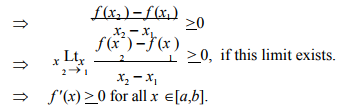Similarly, if f is decreasing on [a,b] then f '(x) ≤ 0, if the derivative exists.

The converse holds with the additional condition, that f is continuous on [a, b].
Note Let f be continuous on [a,b] and has derivative at each point of the open interval (a,b), then
(i) If f '(x) > 0 for every x ∈ (a,b), then f is strictly increasing on [a,b]
(ii) If f '(x) < 0 for every x ∈ (a,b), then f is strictly decreasing on [a,b]
(iii) If f '(x) = 0 for every x ∈ (a,b), then f is a constant function on [a,b]
(iv) If f '(x) > 0 for every x ∈ (a,b), then f is increasing on [a,b]
(v) If f '(x) < 0 for every x ∈ (a,b), then f is decreasing on [a,b]

The above results are used to test whether a given function is increasing or decreasing.

Stationary Value of a Function

A function y = f(x) may neither be an increasing function nor be a decreasing function of x at some point of the interval [a,b]. In such a case, y = f(x) is called stationary at that point. At a stationary point f '(x) = 0 and the tangent is parallel to the x - axis.

Example 1

If y = x- 1/x, prove that y is a strictly increasing function x for all real values of x. (x σ 0)

Solution :
We have y = x -1/x Differentiating with respect to x, we get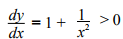for all values of x, except x = 0
∴ y is a strictly increasing function for all real values of x. (x ≠ 0)

Example 2 If y = 1+ 1/x , show that y is a strictly decreasing function x for all real values of x. (x σ 0)
Solution :

We have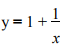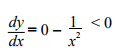for all values of x. (x ≠ 0) dx x 2

∴  y is a strictly decreasing function for all real values of x. (x ≠ 0)

Example 3 Find the ranges of values of x in which 2x3 - 9x2 +12x + 4 is strictly increasing and strictly decreasing.

Solution : Let y = 2x3 - 9x2 + 12x + 4
dx /dy = 6x2 - 18x + 12
= 6(x 2 - 3x + 2)
= 6(x - 2) (x - 1)
dx /dy  > 0 when x < 1 or x > 2
x lies outside the interval (1, 2).
dx /dy   < 0 when 1 < x < 2

∴  The function is strictly increasing outside the interval [1, 2] and strictly decreasing in the interval (1, 2)

Example 4 Find the stationary points and the stationary values of the function f(x) = x3 - 3x2 - 9x + 5. Solution :
Let y = x3 - 3x2 - 9x + 5
dx/dy = 3x 2 - 6x - 9
At stationary points, dy/dx = 0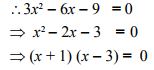The stationary points are obtained
when x = -1 and x = 3
when x = -1, y = (-1)3 - 3(-1)2 - 9(-1) + 5 = 10
when x = 3, y = (3)3 - 3(3)2 - 9(3) + 5 = -22

∴   The stationary values are 10 and -22 The stationary points are (-1, 10) and (3, -22)

Example 5 For the cost function C = 2000 + 1800x - 75x 2 + x 3 find when the total cost (C) is increasing and when it is decreasing. Also discuss the behaviour of the marginal cost (MC)

Solution : Cost function C = 2000 + 1800x  -75x 2 + x 3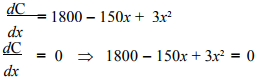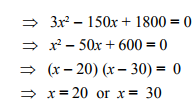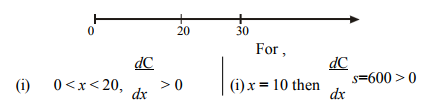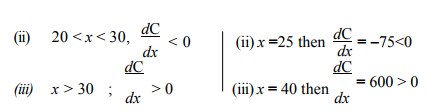∴ C is increasing for 0 < x < 20 and for x > 30. C is decreasing for 20 < x < 30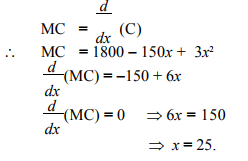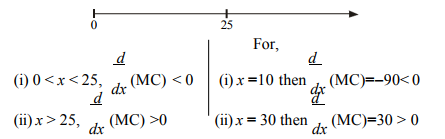∴ MC is decreasing for x < 25 and increasing for x > 25.

Maximum and Minimum Values

Let f be a function defined on [a,b] and c an interior point of [a,b] (i.e.) c is in the open interval (a,b). Then
(i) f(c) is said to be a maximum or relative maximum of the function f at x = c if there is a neighbourhood (c-δ , c + δ) of c such that for all x ∈ (c- δ, c + δ) other than c, f(c) > f(x)
(ii) f(c) issaid to be a minimumorrelativeminimumofthe function f at x = c if there is a neighbourhood (c- δ, c + δ) of c such that for all x ∈ (c- δ, c + δ) other than c, f(c) < f(x).
(iii) f(c) is said to be an extreme value of f or extremum at c if it is either a maximum or minimum.

Local and Global Maxima and Minima

Consider the graph (Fig. 4.1) of the function y = f(x).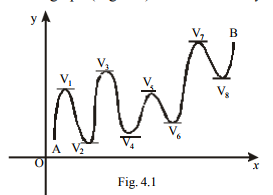The function y = f(x) has several maximum and minimum points. At the points V , V , ...V , dy = 0. In fact the function has 1 2 8 dx maxima at V1 , V3 , V5 , V7 and minima at V2 , V4 , V6 , V8 . Note that maximum value at V5 is less than the minimum value at V8 . These maxima and minima are called local or relative maxima and minima. If we consider the part of the curve between A and B then the function has absolute maximum or global maximum at V7 and absolute minimum or global minimum at V2 .

Note By the terminology maximum or minimum we mean local maximum or local minimum respectively.

Criteria for Maxima and Minima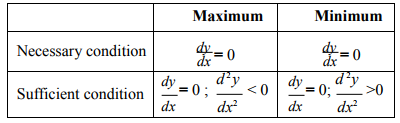Concavity and Convexity
Consider the graph (Fig. 4.2) of the function y = f(x). Let PT be the tangent to the curve y = f(x) at the point P. The curve (or an arc of the curve) which lies above the tangent line PT is said to be concave upward or convex downward.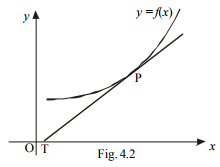The curve (or an arc of the curve) which lies below the tangent line PT (Fig. 4.3) issaid to be convex upward or concave downward.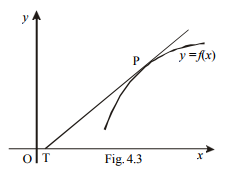Conditions for Concavity and Convexity.

Let f(x) be twice differentiable. Then the curve y = f(x) is (
i) concave upward on any interval if f "(x) > 0
(ii) convex upward on any interval if f "(x) < 0

Point of Inflection A point on a curve y = f(x), where the concavity changes from upto down or vice versa is called a Point of Inflection.

For example, in y = x 1/3 (Fig. 4.4) has a point of inflection at x = 0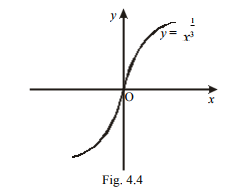Example 6 Investigate the maxima and minima of the function 2x 3 + 3x 2 - 36x + 10. Solution :
Let y = 2x 3 + 3x 2 - 36x + 10 Differentiating with respect to x, we get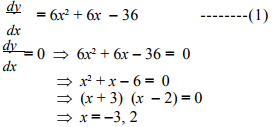Again differentiating (1) with respet to x, we get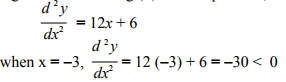∴ It attains maximum at x = -3
∴ Maximum value is y = 2(-3)3 + 3(-3)2 - 36(-3) + 10 = 91 d 2 y
when x =2, dx2 = 12(2) + 6 = 30 > 0
∴ It attains minimum at x = 2
∴ Minimum value is y = 2(2)3 + 3(2)2 - 36(2) + 10 = -34

Example 7
Find the absolute (global) maximum and minimum values of the function f(x) = 3x 5 - 25x 3 + 60x + 1 in the interval [-2, 1]

Solution : Given f(x) = 3x5 - 25x 3 + 60x + 1 f 1 (x) = 15x 4 - 75x 2 + 60

The necessary condition for maximum and minimum is f'(x) = 0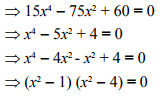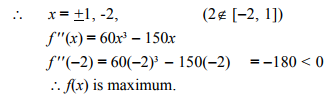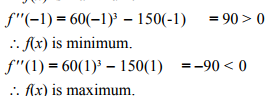The maximum value when x = -2
is f(-2) = 3(-2)5 - 25(-2)3 + 60(-2) + 1 = -15
The minimum value when x = -1
is f(-1) = 3(-1)5 - 25(-1)3 + 60(-1) + 1 = -37
The maximum value when x = 1
is f(1) = 3(1)5 - 25(1)3 + 60(1) + 1 = 39

∴ Absolute maximum value = 39. and Absolute minimum value = -37

Example 8
What is the maximum slope of the tangent to the curve y = -x 3 + 3x 2 +9x - 27 and at what point is it?

Solution : We have y = -x 3 + 3x 2 +9x - 27 Differentiating with respect to x, we get

dx/dy = = -3x 2 + 6x +9
∴  Slope of the tangent is -3x 2 +6x + 9
Let M = -3x 2 +6x + 9

Differenating with respect to x, we get
dM/dx = -6x + 6 ------------(1)

Slope is maximum when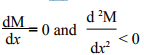dM/dx = 0 ⇒ -6x + 6 = 0
⇒ x = 1

Again differentiating (1) with respect to x, we get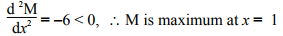∴ Maximum value of
M when x = 1 is M = - 3(1)2 + 6(1)+9 = 12

When x = 1 ; y = - (1)3 +3(1)2 +9(1)- 27 = -16
∴ Maximum slope = 12

The required point is (1, -16)

Example 9
Find the points of inflection of the curve y = 2x 4 - 4x 3 + 3.

Solution

We have y = 2x 4 -4x 3 + 3

Differentiate with respect to x , we get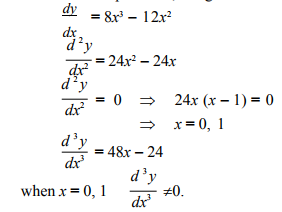∴ points of inflection exist.
when x = 0, y = 2(0)4 - 4(0)3 + 3 = 3
when x = 1, y = 2(1)4 - 4(1)3 + 3 = 1
∴ The points of inflection are (0, 3) and (1, 1)

Example 10
Find the intervals on which the curve f(x) = x 3-6x 2+9x-8 is convex upward and convex downward.

Solution : We have f(x) = x 3 - 6x 2 + 9x - 8

Differentiating with respect o x,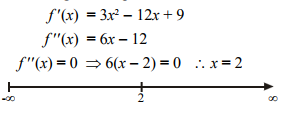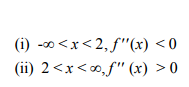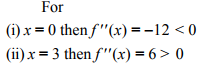∴ The curve is convex upward in the interval (-∞, 2)
The curve is convex downward in the interval (2, ∞)

Offer running on EduRev: Apply code STAYHOME200 to get INR 200 off on our premium plan EduRev Infinity!

122 videos|142 docs

,

,

,

,

,

,

,

,

,

,

,

,

,

,

,

,

,

,

,

,

,

,

,

,

;